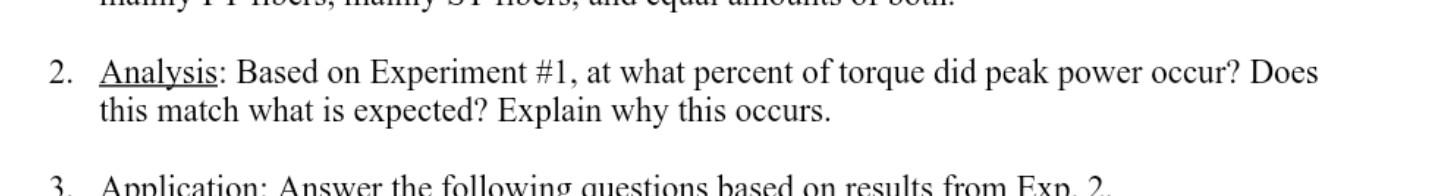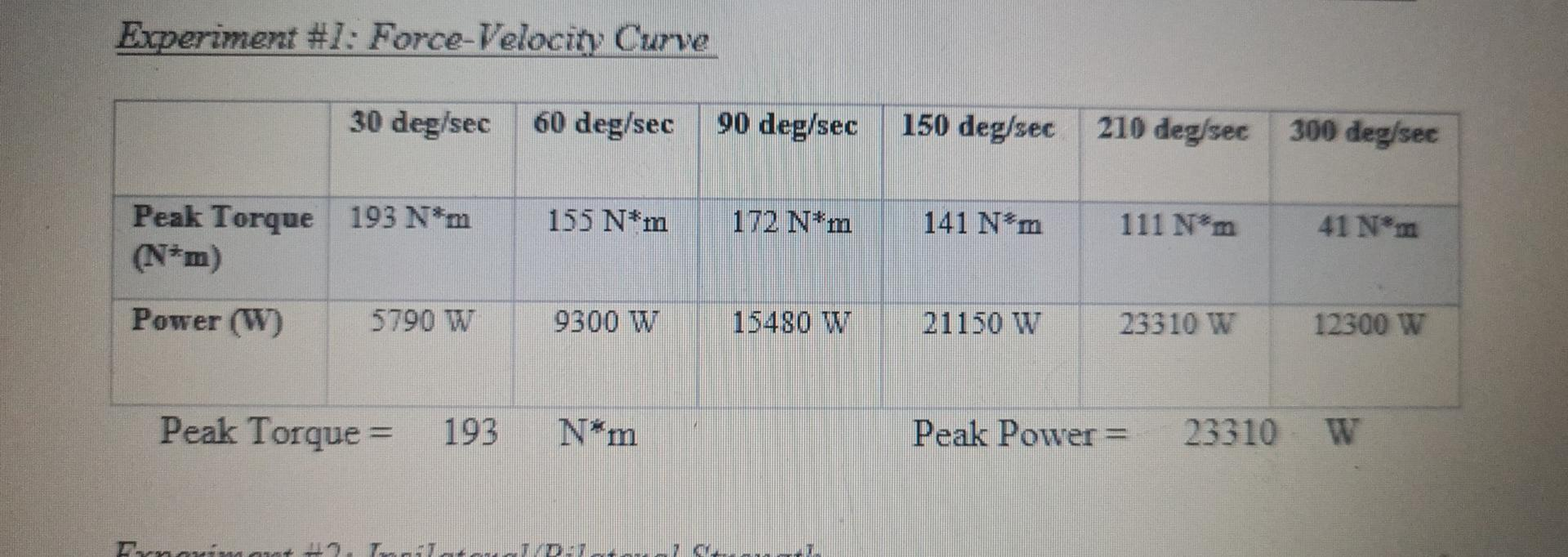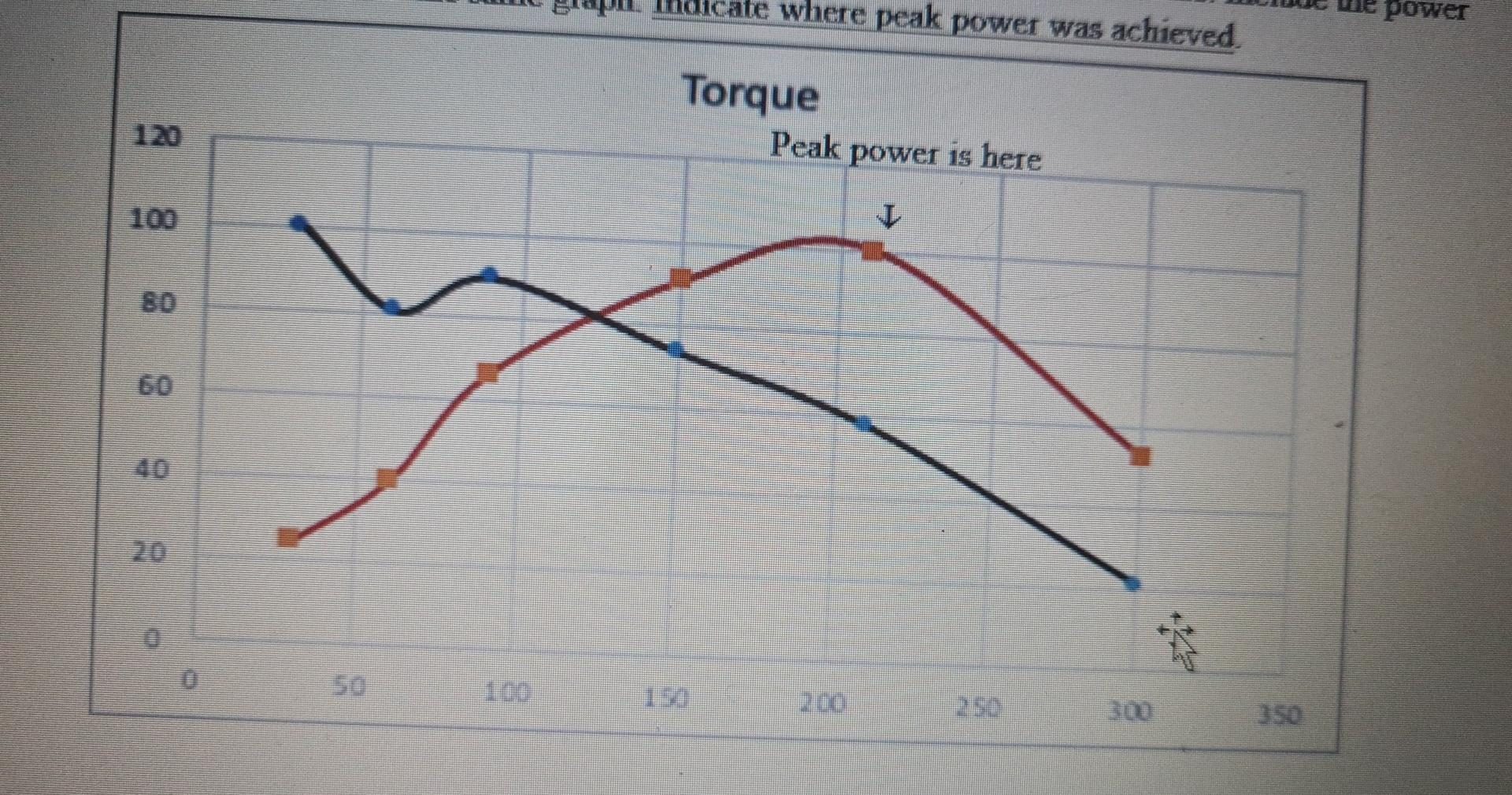# Question peak powe occurred at 100%, I just need to know if its expected and why. 2. Analysis: Based on Experiment #1, at what percent of torque did peak power occur? Does this match what is expected? Explain why this occurs. 3 Application: Answer the following questions based on results from Exp. 2. Experiment #1: Force-Velocity Curve 30 deg/sec 60 deg/sec 90 deg/sec 150 deg/sec 210 deg/sec 300 deg/sec 155 N*m 172 N*m Peak Torque 193 N*m (N*m) 141 N*m 111 Nm 41 N*m Power (W) 5790 W 9300 W 15480 W 21150 W 23310 W 12300 W Peak Torque = 193 N*m Peak Power = 23310 W Trnavim 1. TIT TD ate where peak power was achieved. power Torque Peak power is here IE2LI8B The Asker · Mechanical Engineeringpeak powe occurred at 100%, I just need to know if its expected and why.

Transcribed Image Text: 2. Analysis: Based on Experiment #1, at what percent of torque did peak power occur? Does this match what is expected? Explain why this occurs. 3 Application: Answer the following questions based on results from Exp. 2. Experiment #1: Force-Velocity Curve 30 deg/sec 60 deg/sec 90 deg/sec 150 deg/sec 210 deg/sec 300 deg/sec 155 N*m 172 N*m Peak Torque 193 N*m (N*m) 141 N*m 111 Nm 41 N*m Power (W) 5790 W 9300 W 15480 W 21150 W 23310 W 12300 W Peak Torque = 193 N*m Peak Power = 23310 W Trnavim 1. TIT TD ate where peak power was achieved. power Torque Peak power is here I
More
Transcribed Image Text: 2. Analysis: Based on Experiment #1, at what percent of torque did peak power occur? Does this match what is expected? Explain why this occurs. 3 Application: Answer the following questions based on results from Exp. 2. Experiment #1: Force-Velocity Curve 30 deg/sec 60 deg/sec 90 deg/sec 150 deg/sec 210 deg/sec 300 deg/sec 155 N*m 172 N*m Peak Torque 193 N*m (N*m) 141 N*m 111 Nm 41 N*m Power (W) 5790 W 9300 W 15480 W 21150 W 23310 W 12300 W Peak Torque = 193 N*m Peak Power = 23310 W Trnavim 1. TIT TD ate where peak power was achieved. power Torque Peak power is here I